Courses

# Test: Coordinate Geometry- 4

## 25 Questions MCQ Test UPSC Prelims Paper 2 CSAT - Quant, Verbal & Decision Making | Test: Coordinate Geometry- 4

Description
This mock test of Test: Coordinate Geometry- 4 for UPSC helps you for every UPSC entrance exam. This contains 25 Multiple Choice Questions for UPSC Test: Coordinate Geometry- 4 (mcq) to study with solutions a complete question bank. The solved questions answers in this Test: Coordinate Geometry- 4 quiz give you a good mix of easy questions and tough questions. UPSC students definitely take this Test: Coordinate Geometry- 4 exercise for a better result in the exam. You can find other Test: Coordinate Geometry- 4 extra questions, long questions & short questions for UPSC on EduRev as well by searching above.
QUESTION: 1

### The distance of the point (– 3, 4) from the origin is

Solution:

Let the given point be (x1, y1) = (-3, 4) and the orgin is (x2, y2) = (0, 0)
∴ Distance of the given point from the orgin =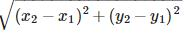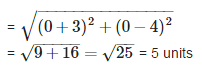QUESTION: 2

### If A and B are the points (– 6, 7) and (– 1, – 5) respectively, then the distance 2AB is equal to 26 units 13 units 15 units 20 units 2AB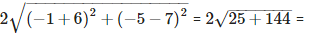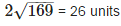Solution: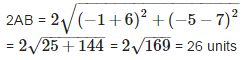QUESTION: 3

### The distance between the points (a, a) and (−√3a,√3a) is

Solution: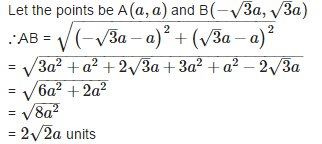QUESTION: 4

Three consecutive vertices of a parallelogram ABCD are A(1, 2), B(1, 0) and C(4, 0). The co – ordinates of the fourth vertex D are

Solution:

Let coordinates of D be (x, y).
Since diagonals of a parallelogram bisect each other.

Therefore, coordinates of O will be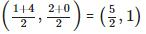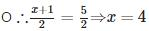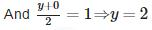Therefore, the required coordinates are (4, 2).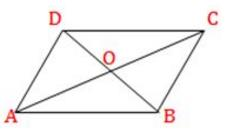QUESTION: 5

If (a, 0) , (0, b) and (x, y) are collinear, then

Solution:

If given points are collinear, the triangle formed by these three points is 0.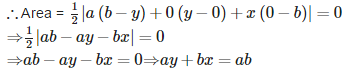QUESTION: 6

The distance between the points (– 1, – 5) and (– 6, 7) is

Solution:

Let point A be (x1, y1) = (-1, -5) and point B (x2, y2) = (-6, 7)
∴Distance between A and B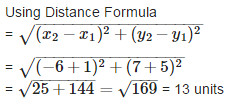QUESTION: 7

If P(x, y) is any point on the line joining the points A(a, 0) and B(0, b), then

Solution: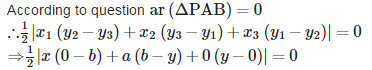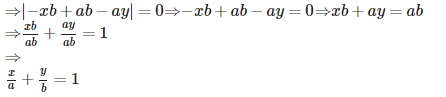QUESTION: 8

The distance between the points A(p sin 25, 0) and B(0, p sin 65) is

Solution:

p units p2 units 1 units 0 units Distance between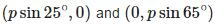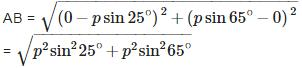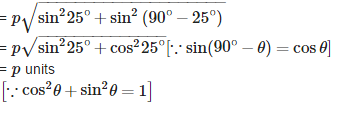QUESTION: 9

The length of the median through A of  ΔABC with vertices A(7, – 3), B(5, 3) and C(3, – 1) is

Solution: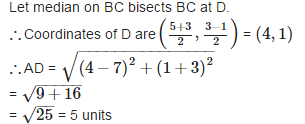QUESTION: 10

If the co – ordinates of a point are (3, – 7), then its ordinate is

Solution:

Since y−coordinate is called ordinate.
Therefore, ordinate is −7.

QUESTION: 11

The distance between the points (a, b) and (– a, b) is

Solution:

Let point A be (a, b) and point B is (-a, b)

∴ distance between A and B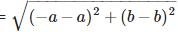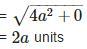QUESTION: 12

The vertices of a quadrilateral are (1, 7), (4, 2), (– 1, – 1) and (– 4, 4). The quadrilateral is a

Solution:

Let A (1, 7), B (4, 2), C (-1, -1) and D(-4, 4) are the vertices of a quardinate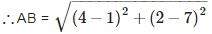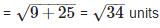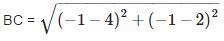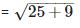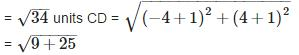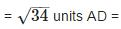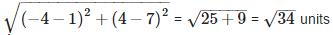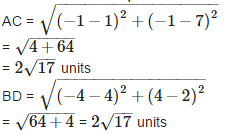Since, all sides are equal and both diagonals are also equal.
Therefore, the given quadrilateral is a square.

QUESTION: 13

The distance between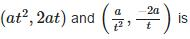Solution:

The distance between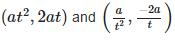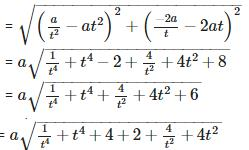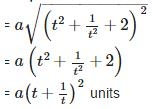QUESTION: 14

If the line segment joining the points A(x1,y1) and B(x2,y2) is divided by a point P in the ratio 1 : k internally, then the co – ordinates of the point P are

Solution: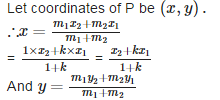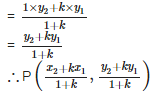QUESTION: 15

If the co – ordinates of a point are (– 5, 11), then its abscissa is

Solution:

Since x−coordinate is called abscissa.
Therefore, abscissa is −5.

QUESTION: 16

If the distance between the points (p, – 5) and (2, 7) is 13 units, then the value of ‘p’ is

Solution:

Let point A be (p,−5)(p,−5) and point B (2, 7) and distance between A and B = 13 units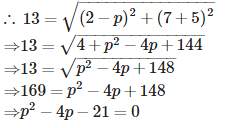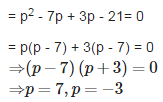QUESTION: 17

If A is point on the x – axis whose abscissa is 5 and B is the point (1, – 3), then the distance AB is

Solution: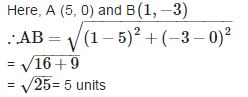QUESTION: 18

The point where the medians of a triangle meet is called the ________ of the triangle

Solution:

The point where the medians of a triangle meet is called thecentroidof the triangle.

QUESTION: 19

The base of an equilateral triangle ABC lies on the y – axis. The co – ordinates of the point C is (0, – 3). If origin is the midpoint of BC, then the co – ordinates of B are

Solution: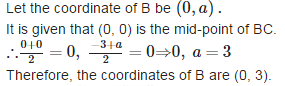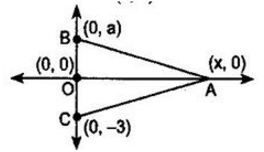QUESTION: 20

The abscissa of any point on the y – axis is

Solution:

Since coordinates of any point on y−axis is (0,y).
Therefore, abscissa is 0.

QUESTION: 21

The values of ‘y’ for which the distance between the points (2, – 3) and (10, y) is 10 units is

Solution:

Let  one point be A (2,−3) and 2nd point is B (10,y) and

distance between point A and point B = AB = 10 units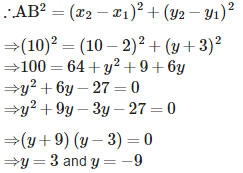QUESTION: 22

The distance of the point (– 5, 12) from the y – axis is

Solution:

The distance of any point from y−axis is its abscissa. Therefore, the required distance is 5 units.

QUESTION: 23

The co – ordinates of the mid – point of the line segment joining the points (– 2, 3) and (4, – 5) are

Solution:

Let the coordinates of midpoint C(x,y) of the line segment joining the points A(−2,3) and B(4,−5).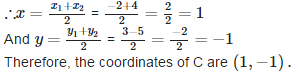QUESTION: 24

The point where the perpendicular bisector of the line segment joining the points A(2, 5) and B(4, 7) cuts is:

Solution:

Since, the point, where the perpendicular bisector of a line segment cuts, is the mid-point of that line segment.
∴ Coordinates of Mid-point of line segment AB =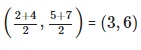QUESTION: 25

The distance of a point from the y – axis is called

Solution:

The distance of a point from the y – axis is called abscissa.